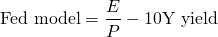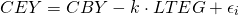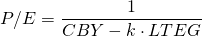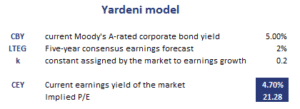# Yardeni model

The Yardeni model is a valuation model used to determine whether the overall stock market is overvalued or not. The model is an extension of the Fed model. Therefore, to properly discuss the model we first need to discuss the Fed model. Common examples of stock market indices to which the Yardeni model is applied are the S&P 500 and Dow Jones Industrial Average (DJIA). To assess whether or not stock markets are overvalued, investors often calculate the Yardeni-implied price-earnings ratio (P/E).

On this page, we discuss the Fed model, the Yardeni model definition and formula as well as an example that illustrates how to calculate the P/E using the model. The Excel example can be downloaded at the bottom of the page.

## Fed model

First, we start with a brief discussion of the Fed model. The Fed model considers the overall market to be overvalued if the earnings yield on the S&P 500 is lower than the yield on a 10Y U.S. bond. Similarly, the S&P 500 is undervalued if the E/P is higher than the 10Y yield. Using a formulawe can easily determine whether or not the equity market is overvalued. In particular, if the result is negative (positive), then the equity market overvalued (undervalued).

## Yardeni model

The Yardeni model starts from the above Fed model. It tries to improve the above approach by including the expected earnings growth rate in the analysiswhere CEY is the current earnings yield of the market, CBY is the current Moody’s A-rated corporate bond yield, LTEG is the five-year consensus earnings growth rate, and k is constant assigned by the market to earnings growth (+/- 0.2).

We can take the reciprocal to obtain the P/E ratio## Yardeni model interpretation

The model indicates that the P/E ratio of the market is negatively related to interest rates and positively related to growth. The following table applies the model to actual data. The spreadsheet used is available for download at the bottom of the page.## Summary

We discussed the valuation model proposed by Yardeni. It is a valuation model used to determine whether or not the aggregate stock market is overvalued. It is often used by analysts to calculate the market implied price-earnings multiple.

### Calculation

Want to have an implementation in Excel? Download the Excel file: Yardeni